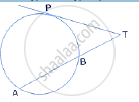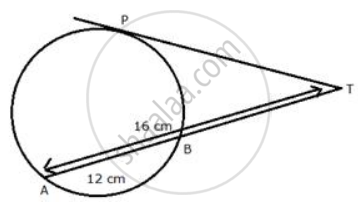Share

# In the Given Figure, Find Tp If at = 16 Cm and Ab = 12 Cm. - Mathematics

Course

#### Question

In the given figure, find TP if AT = 16 cm and AB = 12 cm.#### SolutionPT is the tangent and TBA is the secant of the circle.

Therefore, TP2 = TA x TB

TP2 = 16 × (16 − 12) = 16 × 4 = 64 = (8)2

Therefore, TP = 8 cm

Is there an error in this question or solution?